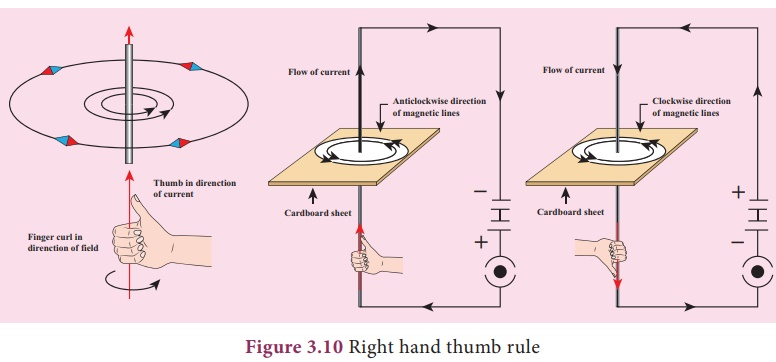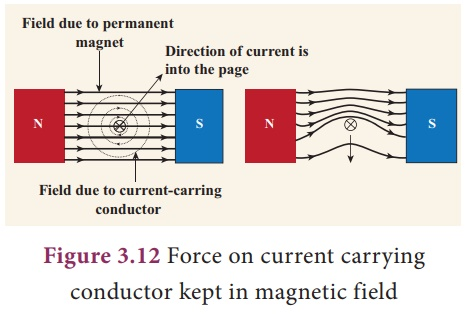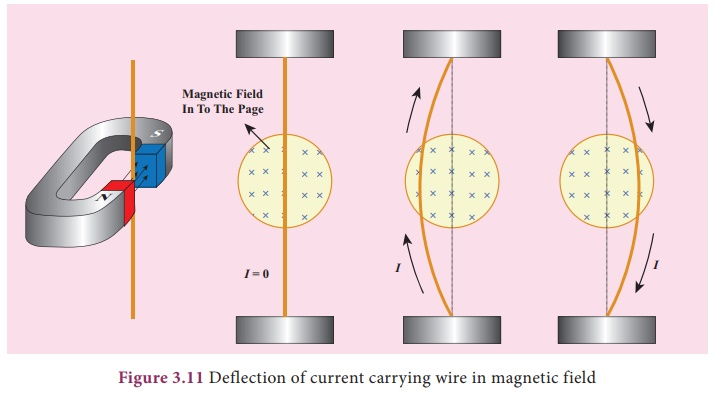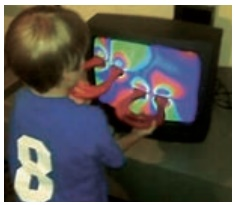Home | | Science 9th std | Force on a current carrying conductor in a magnetic field

# Force on a current carrying conductor in a magnetic field

H.A.Lorentz found that a charge moving in a magnetic field, in a direction other than the direction of magnetic field, experiences a force. It is called the magnetic Lorentz force.

Force on a current carrying conductor in a magnetic field

H.A.Lorentz found that a charge moving in a magnetic field, in a direction other than the direction of magnetic field, experiences a force. It is called the magnetic Lorentz force. Since charge in motion constitutes a current, a conductor carrying moving charges, placed in magnetic field other than the direction of magnetic field, will also experience a force and can produce motion in the conductor.In activity 3, we saw that a current carrying wire has a magnetic field perpendicular to the wire, by looking at the deffection of the compass needle in the vicinity of a current carrying conductor. The deffection of the needle implies that the current carrying conductor exerts a force on the compass needle. In 1821, Michael Faraday discovered that a current carrying conductor also gets deffected when it is placed in a magnetic field. In Figure 3.11, we can see that the magnetic field of the permanent magnet and the magnetic field produced by the current carrying conductor interact and produce a force on the conductor. The view perpendicular to the direction of current is shown in Figure 3.12.If a current I is flowing through a conductor of length L kept perpendicular to the magnetic field B, then the force F experienced by it is given by the equation,

F = I L B

The above equation indicates that the force is proportional to current through the conductor, length of the conductor and the magnetic field in which the current carrying conductor is kept.Note : The angle of inclination between the current and magnetic field also a ects the magnetic force. When the conductor is perpendicular to the magnetic field, the force will be the maximum (=BIL). When it is parallel to the magnetic field, the force will be zero.

The force is always a vector quantity. A vector quantity has both magnitude and direction. It means we should know the direction in which the force would act. The direction is often found using what is known as Flemingâ€™s Left hand Rule (formulated by the scientist John Ambrose Fleming).

The law states that while stretching the three ngers of le hand in perpendicular manner with each other, if the direction of the current is denoted by middle nger of the le hand and the second nger is for direction of the magnetic field then the thumb of the le hand denotes the direction of the force or movement of the conductor (Fig. 3.13)### Exercise 1

A conductor of length 50 cm carrying a current of 5 A is placed perpendicular to a magnetic field of induction 2Ă—10â€“3 T. Find the force on the conductor.

### Solution:

Force on the conductor = I L B

= 5 Ă— 50 Ă— 10â€“2 Ă— 2 Ă—10â€“3

= 5 Ă— 10â€“3 N

### Exercise 2

A current carrying conductor of certain length, kept perpendicular to the magnetic field experiences a force F. What will be the force if the current is increased four times, length is halved and magnetic field is tripled?

### Solution:

Let the current be I, length be L, and magnetic field be B. Therefore, F = I L B.

When current is increased four times, length is halved and magnetic field tripled then, force,

F1 = (4I) x ( L/2) x (3 B)

F1 = 6 F

Therefore, the force increases six times.

Activity 3

Take a cardboard and thread a wire perpendicular through it. Connect the wire such that current flows up the wire. Switch on the circuit. Let the current flow. Place a magnetic compass on the cardboard. Mark S and N point of the compass as X and Y respectively on the cardboard. Move the compass such that S end touches Y. Now mark the N end as Z. In the next step move the compass such that S end touch Z. Repeat the steps. Now if you join all the points you will find that it is a circle. Start again, but now keep the compass away from the center or towards the center. If you follow the above steps, you can see that you can draw another magnetic line and the magnetic lines are concentric circles. Also you will find the magnetic lines are anti-clockwise.Reverse the direction of the current, you will find the magnetic circles are clockwise. Note: The flow of current here means conventional current and not the direction of the flow of electrons.

Activity 4

Stand near the TV screen ( The old CRT type TV). Do you feel any sensation on your skin? Take a bar magnet and bring it near the TV Screen. What do you observe? You can observe that the picture on the screen is distorted. Move the bar magnet away from the screen. Now you will get a clear picture. Repeat this to con rm that the motion of electrons is a ected by the eld produced by the bar magnet. is must be due to the fact that the magnetic eld exerts force on the moving charges. is force is called magnetic force.Michael Faraday (22 September 1791 â€“ 25 August 1867) was a British Scientist who contributed to the study of electromagnetism and electrochemistry.His main discoveries include the principles underlying electromagnetic induction, diamagnetism and electrolysis.

Although Faraday received little formal education, he was one of the most in uential scientists in history. Faraday was an excellent experimentalist who conveyed his ideas in clear and simple language. e SI unit of capacitance is named in his honour: the farad. Albert Einstein kept a picture of Faraday on his study wall, alongside pictures of Isaac Newton and James Clerk Maxwell. Faraday is one of the greatest scienti c discoverers of all time.

Study Material, Lecturing Notes, Assignment, Reference, Wiki description explanation, brief detail
9th Science : Magnetism and Electromagnetism : Force on a current carrying conductor in a magnetic field |